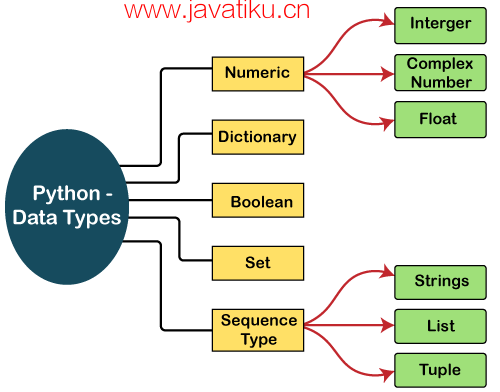# Python教程-Python 数据类型``a = 5``

``````a=10
b="Hi Python"
c = 10.5
print(type(a))
print(type(b))
print(type(c)) ``````

``````<type 'int'>
<type 'str'>
<type 'float'>``````

## 标准数据类型

Python 指定了每种标准数据类型的存储方法。以下是 Python 定义的数据类型列表。

1. 数字（Numbers）
2. 序列类型（Sequence Type）
3. 布尔值（Boolean）
4. 集合（Set）
5. 字典（Dictionary）### 数字（Numbers）

``````a = 5
print("The type of a", type(a))

b = 40.5
print("The type of b", type(b))

c = 1+3j
print("The type of c", type(c))
print(" c is a complex number", isinstance(1+3j,complex))  ``````

``````The type of a <class 'int'>
The type of b <class 'float'>
The type of c <class 'complex'>
c is complex number: True``````

Python 支持三种类型的数值数据。

• 整数（Int）： 整数值可以是任何长度，如 10、2、29、-20、-150 等。在 Python 中，整数可以是任何您想要的长度。它的值属于 int 类型。
• 浮点数（Float）： 浮点数存储浮点数，如 1.9、9.902、15.2 等。它可以精确到小数点后 15 位。
• 复数（Complex）： 复数包含一个有序对，即 x + iy，其中 x 和 y 分别表示实部和虚部。复数例如 2.14j、2.0 + 2.3j 等。

### 字符串（String）

``````str = "string using double quotes"
print(str)
s = '''''A multiline
string'''
print(s)  ``````

``````string using double quotes
A multiline
string``````

``````str1 = 'hello javatpoint' #string str1
str2 = ' how are you' #string str2
print (str1[0:2]) #printing first two character using slice operator
print (str1) #printing 4th character of the string
print (str1*2) #printing the string twice
print (str1 + str2) #printing the concatenation of str1 and str2   ``````

``````he
o
hello javatpointhello javatpoint
hello javatpoint how are you``````

### 列表（List）

``````list1  = [1, "hi", "Python", 2]
#Checking type of given list
print(type(list1))

#Printing the list1
print (list1)

# List slicing
print (list1[3:])

# List slicing
print (list1[0:2])

# List Concatenation using + operator
print (list1 + list1)

# List repetation using * operator
print (list1 * 3)  ``````

``````[1, 'hi', 'Python', 2]

[1, 'hi']
[1, 'hi', 'Python', 2, 1, 'hi', 'Python', 2]
[1, 'hi', 'Python', 2, 1, 'hi', 'Python', 2, 1, 'hi', 'Python', 2]``````

### 元组（Tuple）

``````tup  = ("hi", "Python", 2)
# Checking type of tup
print (type(tup))

#Printing the tuple
print (tup)

# Tuple slicing
print (tup[1:])
print (tup[0:1])

# Tuple concatenation using + operator
print (tup + tup)

# Tuple repatation using * operator
print (tup * 3)

# Adding value to tup. It will throw an error.
t = "hi"  ``````

``````<class 'tuple'>
('hi', 'Python', 2)
('Python', 2)
('hi',)
('hi', 'Python', 2, 'hi', 'Python', 2)
('hi', 'Python', 2, 'hi', 'Python', 2, 'hi', 'Python', 2)

Traceback (most recent call last):
File "main.py", line 14, in <module>
t = "hi";
TypeError: 'tuple' object does not support item assignment``````

### 字典（Dictionary）

``````d = {1:'Jimmy', 2:'Alex', 3:'john', 4:'mike'}

# Printing dictionary
print (d)

# Accesing value using keys
print("1st name is "+d)
print("2nd name is "+ d)

print (d.keys())
print (d.values())    ``````

``````1st name is Jimmy
2nd name is mike
{1: 'Jimmy', 2: 'Alex', 3: 'john', 4: 'mike'}
dict_keys([1, 2, 3, 4])
dict_values(['Jimmy', 'Alex', 'john', 'mike'])``````

### 布尔值（Boolean）

``````# Python program to check the boolean type
print(type(True))
print(type(False))
print(false) ``````

``````<class 'bool'>
<class 'bool'>
NameError: name 'false' is not defined``````

### 集合（Set）

Python 集合是无序的数据类型。它是可迭代的、可变的（创建后可更改）并且具有独特的元素。集合的元素没有固定的顺序；它可能返回元素的不同顺序。要创建集合，可以通过花括号和逗号传递元素序列，也可以使用内置函数 set() 来创建集合。它可以包含不同类型的值。

``````# Creating Empty set
set1 = set()

set2 = {'James', 2, 3,'Python'}

#Printing Set value
print(set2)

# Adding element to the set

``````{3, 'Python', 'James', 2}